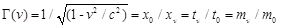Blog

To achieve interstellar travel, the Kline Directive instructs us to be bold, to explore what others have not, to seek what others will not, to change what others dare not. To extend the boundaries of our knowledge, to advocate new methods, techniques and research, to sponsor change not status quo, on 5 fronts, Legal Standing, Safety Awareness, Economic Viability, Theoretical-Empirical Relationships, and Technological Feasibility.

In this post I discuss three concepts, that if implemented should speed up the rate of innovation and discovery so that we can achieve interstellar travel within a time frame of decades, not centuries.

Okay, what I’m going to say will upset some physicists, but I need to say it because we need to resolve some issues in physics to distinguish between mathematical construction and conjecture. Once we are on the road to mathematical construction, there is hope that this will eventually lead to technological feasibility. This post is taken from my published paper “Gravitational Acceleration Without Mass And Noninertia Fields” in the peer reviewed AIP journal, Physics Essays, and from my book An Introduction to Gravity Modification.

The Universe is much more consistent than most of us (even physicists) suspect. Therefore, we can use this consistency to weed out mathematical conjecture from our collection of physical hypotheses. There are two set of transformations that are observable. The first, in a gravitational field at a point where acceleration is a compared to a location at 0 an infinite distance from the gravitational source, there exists Non-Linear transformations Γ(a) which states that time dilation ta/t0, length contraction x0/xa, and mass increase ma/m0, behave in a consistent manner such that:(1)

.

The second consistency is Lorentz-Fitzgerald transformations Γ(v) which states that at a velocity v compared to rest at 0, time dilation tv/t0, length contraction x0/xv, and mass increase mv/m0, behave in a consistent manner such that:(2)

.

Now here is the surprise. The Universe is so consistent that if we use the Non-Linear transformation, equation (1) to calculate the free fall velocity (from infinity) to a certain height above the planet’s or star’s surface, and it’s corresponding time dilation, we find that it is exactly what the Lorentz-Fitzgerald transformation, equation (2) requires. That there is this previously undiscovered second level of consistency!

You won’t find this discovery in any physics text book. Not yet anyway. I published this in my 2011 AIP peer reviewed Physics Essays paper, “Gravitational Acceleration Without Mass And Noninertia Fields”.

Now let us think about this for a moment. What this says is that the Universe is so consistent that the linear velocity-time dilation relationship must be observable where ever velocity and time dilation is present, even in non-linear spacetime relationships where acceleration is present and altering the velocity and therefore the time dilation present.

Or to put it differently, where ever Γ(a) is present the space, time, velocity and acceleration relationship must allow for Γ(v) to be present in a correct and consistent manner. When I discovered this I said, wow! Why? Because we now have a means of differentiating hypothetical-theoretical gravitational fields, and therefore mathematical conjectures, from natural-theoretical gravitational fields, which are correct mathematical constructions.

That is, we can test the various quantum gravity & string hypotheses and any of the tensor metrics! Einstein’s tensor metrics should be correct, but from a propulsion perspective there is something more interesting, Alcubierre tensor metrics. Alcubierre was the first, using General Relativity, to propose the theoretical possibility of warp speed (note, not how to engineer it). Alcubierre’s work is very sophisticated. However, the concept is elegantly simple. That one can wrap a space craft in gravitational-type deformed spacetime to get it to ‘fall’ in the direction of travel.

The concept suggest that both equations (1) and (2) are no longer valid as the relative velocity between the outer edges of the spacetime wrap and an external observer is either at c, the velocity of light or greater – one needs to do the math to get the correct answer. Even at an acceleration of 1g, and assuming that this craft has eventually reached c, equation (1) and (2) are no longer consistent. Therefore, my inference is that Alcubierre metrics allows for zero time dilation within the wrap but not velocities greater than the velocity of light. Therefore, it is also doubtful that Dr. Richard Obousy hypothesis that it is possible to achieve velocities of 1E30c with a quantum string version of Alcubierre warp drive is correct.

—————————————————————————————————

Benjamin T Solomon is the author & principal investigator of the 12-year study into the theoretical & technological feasibility of gravitation modification, titled An Introduction to Gravity Modification, to achieve interstellar travel in our lifetimes. For more information visit iSETI LLC, Interstellar Space Exploration Technology Initiative.

Solomon is inviting all serious participants to his LinkedIn Group Interstellar Travel & Gravity Modification.